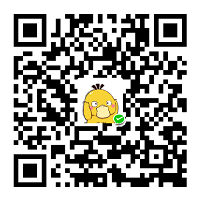Python中，空列表，空元祖和空字典，以及未初始化的类对象都被Python判断为False，所以可以通过if not的方式判断这些对象是否为空。

1 Python类对象判空

# -*- coding: UTF-8 –*-

class BasicObject:
m_Value = 0

def __init__(self):
self.m_Value = 100

if __name__ == '__main__':
# 类对象判空
# 不初始化
basic = None
if not basic:
print("对象为空")
else:
print("对象不为空")

# 初始化
basic = BasicObject()
if not basic:
print("对象为空")
else:
print("对象不为空,m_Value的值为{}".format(basic.m_Value))

2 Python列表判空

2.1 方法1

# -*- coding: UTF-8 –*-

if __name__ == '__main__':

A = []
if not A:
print("列表为空")
else:
print("列表不为空")

A = ['1','2']
if not A:
print("列表为空")
else:
print("列表不为空")

3 Python元祖判空

3.1 方法1

# -*- coding: UTF-8 –*-

if __name__ == '__main__':

A = ()
if not A:
print("元祖为空")
else:
print("元祖不为空")

A = ('1',2)
if not A:
print("元祖为空")
else:
print("元祖不为空")

4 Python字典判空

4.1 方法1

# -*- coding: UTF-8 –*-

if __name__ == '__main__':

A = {}
if not A:
print("字典为空")
else:
print("字典不为空")

A = {
'name' : 'liming',
'age' : 18
}
if not A:
print("字典为空")
else:
print("字典不为空")支付宝扫一扫微信扫一扫纵使晴明无雨色，入云深处亦沾衣。